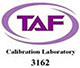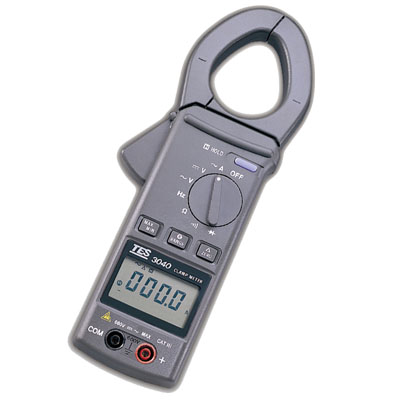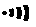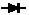•• 繁體
• English

## 钩表

DC/AC Current Clamp MeterTES-3040H

## 数位钩表

• 3-3/4 位LCD含模拟条形码显示
• 自动换文件于电压、奥姆及频率测量
• 自动关机功能
• 数据锁定功能
• 最大值/最小值记录功能
• 零调/相对值功能

测量规格：

`    • 交流电流ACA :自动 / 手动选档 (低通滤波器) `
 `范围` `分辨率` `准确度` `过载保护` `频率响应` `400A` `0.1A` `±(2%+10)` `1100A` `40～450 Hz` `1000A` `1A`
`  `
• `直流电压DCV：自动 / 手动选档 `
 `范围` `分辨率` `准确度` `输入阻抗` `过载保护` `400mV` `0.1 mV` `±(0.75%+2)` `≒ 11MΩ` `AC / DC 600V` `4V` `0.001V` `≒ 10MΩ` `40V` `0.01V` `400V` `0.1V` `600V` `1V`
`  `
• `交流电压ACV：自动 / 手动选档 (低通滤波器) `
 `范围` `分辨率` `准确度` `输入阻抗` `过载保护` `频率响应` `4V` `0.001V` `±(1.2%+5)` `≒ 11MΩ` `AC / DC 600V` 40 ～450 Hz `40V` `0.01V` `≒ 10MΩ` `400V` `0.1V` `600V` `1V` `±(1.5%+5)`
`  `
• `电阻Ω：自动 / 手动选档 `
 `范围` `分辨率` `准确度` `最大开路电压` `过载保护` `400Ω` `0.1Ω` `±( 1﹪+5)` `≦ 0.5 VDC` `600Vrms` `4KΩ` `0.001KΩ` `±( 1﹪+3)` `40KΩ` `0.01KΩ` `400KΩ` `0.1KΩ` `4000KΩ` `1KΩ` `40MΩ` `0.01MΩ` `±( 1.5﹪+3)`
`  `
• `电压频率测量Hz：自动选档 `
 `范围` `分辨率` `准确度` `灵敏度` `过载保护` `100Hz` `0.01Hz` `±( 0.1﹪+2)` `100mV` `600Vrms` `1KHz` `0.1Hz` `5KHz` `0.001KHz`
`  `
• `电流频率测量Hz：自动选档 `
 `范围` `分辨率` `准确度` `灵敏度` `过载保护` `100Hz` `0.01Hz` `±( 0.5﹪+5)` `5A` `1100A` `1KHz` `0.1Hz`
`  `
• `导通测量 `
 `范围` `动作范围` `最大开路电压` `过载保护` `` `≦ 40Ω` `≦ 0.5V` `600Vrms`
`  `
• `二极管测量 `
 `范围` `分辨率` `准确度` `最大测试电流` `最大开路电压` `过载保护` `` `0.001V` `±(1%+2)` `1.0mA` `3.2V` `600Vrms`

"哔哔" 声之连续警告声。

附件: 说明书、电池、测试棒、皮套。